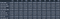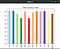Photo by Charles Deluvio on Unsplash

# Considerations that must be made before choosing a machine learning algorithm

“What machine learning algorithm should I use? “ or “How to choose a machine learning algorithm?” Let’s start with: there is no straightforward answer! 😤 😕 however, there are some factors that clearly influence the choice of an algorithm.

One of the key decisions that typically a data scientist has to make is the choice of an algorithm that will use the data to hopefully predict correctly the expected outcome. However, faced with a lot of algorithms, data scientists find themselves overwhelmed. Despite the fact that there are no direct and straightforward answers to the question “Which algorithm will I choose”, there are some tips that can make this decision easier. In this article, I will try to explain them.

Deciding the machine learning algorithm is part of an ML project workflow. But, before you start testing different ML algorithms, you need to go through the other phases of the workflow in order to have a clear understanding of your data and your problem. This is probably the most critical part. Having a clear understanding of your data will help you make good and better decisions along your project.

I will divide the factors into 4 main points: the data, time, metrics and a bonus

# The Data

“What are the key characteristics of my data?”

With this in mind you can analyze different characteristics:

1. Size of the data:
• Direct tips: for small datasets, you will want to try algorithms with low variance and high bias. On the other hand, algorithms with high variance and low bias will work better for large datasets.
• So, in the first case, you will choose algorithms like Linear Regression, Naive Bayes or Linear SVM. And for the second case, you will want to test algorithms like KNN, Decision Trees or Kernel SVM

2. Linearity:

• Here the question is simple: “Is the data linear?” or “ Is the data linearly separable?”
• If the data can be separated by a straight line (or other higher-dimensional “lines”) you can use algorithms like Linear Regression, Logistic Regression or SVM. These algorithms assume that the data is linear and are a good option because of their low complexity
• On the other hand, if the data is not linear we will have to opt for algorithms that can handle with complex and high dimensional data structures. Some examples are Random Forest, Kernel SVM or neural networks

# Speed (training time and prediction time)

Training time:

• Question: How long does it take to build, train, and test the model?
• Sometimes we don’t have a lot of time to train and want to test fast an assumption. In these cases, it’s good to choose an ML algorithm that takes less time to train. Examples include KNN, Logistic Regression, Linear Regression and Naive Bayes. These algorithms are easy to implement and quick to run.
• If we have more time, we chan choose algorithms that involve tuning more parameters like SVM, Neural Networks or Random Forest

Prediction time:

• Question. How long does it take to make predictions?
• In some projects, we want to be able to get fast predictions of our live data. For example, stock market price prediction. In these cases, it makes sense to choose an algorithm that can make quick predictions like SVM, Logistic Regression and linear regression.
• If we don’t have this time constraint, we can also test and use some algorithms that take longer, for example, ensemble models.

# Metrics

Of course, there are a lot of metrics and each metric can tell you something about the model performance. That’s why you have to understand your problem and decide the metrics that will help you define the success of your project. Depending on the type of problem (classification or regression) and what you want to maximize/minimize (False Positives, False negatives, etc) you will choose the appropriate metrics.

# Bonus

As you can see in the two following images, it is possible to compare the results of multiple algorithms and then choose the best algorithmOverall results of 10 algorithmsMean accuracy for each of the tested classifiers

# Conclusion / Summary

• You really have to understand your problem, your data and your constraints in order to select a handful of algorithms
• Try multiple algorithms and compare their performances (for example, using the provided code — https://github.com/FilipeGood/Evaluate-ML-Algorithms)
• Despite the fact that having a good algorithm is important, having a good dataset is even more important!

Questions:

• What is the size of my data?
• Is my data linear?
• How much time do I have to train?
• How quickly do I want my model to make predictions?
• Which metrics will determine if my model is good or not?

Big Data Engineer & AI Master’s Degree Student linkedin.com/in/filipe-good

## More from Filipe Good

Big Data Engineer & AI Master’s Degree Student linkedin.com/in/filipe-good